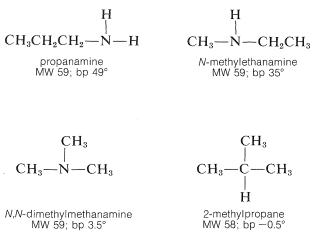# 23.4: Physical Properties of Amines

$$\newcommand{\vecs}{\overset { \rightharpoonup} {\mathbf{#1}} }$$ $$\newcommand{\vecd}{\overset{-\!-\!\rightharpoonup}{\vphantom{a}\smash {#1}}}$$$$\newcommand{\id}{\mathrm{id}}$$ $$\newcommand{\Span}{\mathrm{span}}$$ $$\newcommand{\kernel}{\mathrm{null}\,}$$ $$\newcommand{\range}{\mathrm{range}\,}$$ $$\newcommand{\RealPart}{\mathrm{Re}}$$ $$\newcommand{\ImaginaryPart}{\mathrm{Im}}$$ $$\newcommand{\Argument}{\mathrm{Arg}}$$ $$\newcommand{\norm}{\| #1 \|}$$ $$\newcommand{\inner}{\langle #1, #2 \rangle}$$ $$\newcommand{\Span}{\mathrm{span}}$$ $$\newcommand{\id}{\mathrm{id}}$$ $$\newcommand{\Span}{\mathrm{span}}$$ $$\newcommand{\kernel}{\mathrm{null}\,}$$ $$\newcommand{\range}{\mathrm{range}\,}$$ $$\newcommand{\RealPart}{\mathrm{Re}}$$ $$\newcommand{\ImaginaryPart}{\mathrm{Im}}$$ $$\newcommand{\Argument}{\mathrm{Arg}}$$ $$\newcommand{\norm}{\| #1 \|}$$ $$\newcommand{\inner}{\langle #1, #2 \rangle}$$ $$\newcommand{\Span}{\mathrm{span}}$$$$\newcommand{\AA}{\unicode[.8,0]{x212B}}$$

The physical properties of amines depend in an important way on the extent of substitution at nitrogen. Thus primary amines, $$\ce{RNH_2}$$, and secondary amines, $$\ce{R_2NH}$$, are less volatile than hydrocarbons of similar size, weight, and shape, as the following examples show:This is because the amines are associated through hydrogen bonding of the type $$\ce{N-H} \cdots \colon \ce{N}$$. Generally, $$\ce{N-H} \cdots \colon \ce{N}$$ bonds are somewhat weaker than those of the corresponding types, $$\ce{O-H} \cdots \colon \ce{O}$$ and $$\ce{F-H} \cdots \colon \ce{F}$$, because the electronegativity of nitrogen is less than that of oxygen or fluorine thereby making nitrogen a poorer hydrogen donor. Even so, association through hydrogen bonding is significant in amines of the type $$\ce{RNH_2}$$ or $$\ce{R_2NH}$$ as the boiling-point comparison shows. With tertiary amines, where $$\ce{N-H} \cdots \colon \ce{N}$$ bonding is not possible, the boiling points are much lower and are similar to those of hydrocarbons of similar branching and molecular weights:The water solubilities of the lower-molecular-weight amines are appreciable, as can be seen from the solubility data in Table 23-1. In fact, amines are more water-soluble than alcohols of similar molecular weights. This is the result of hydrogen bonding, with amine molecules as the hydrogen acceptors and water molecules as the hydrogen donors:Hydrogen bonds of this type are stronger than $$\ce{O} \colon \cdots \ce{H-O-H}$$ bonds.

Amines, especially those with significant volatility, have unpleasant odors. Some of them smell like ammonia, others smell fishy, while others are indescribably revolting. The alkanediamines of structure $$\ce{H_2N(CH_3)}_n \ce{NH_2}$$ are notably wretched and two are aptly called putrescine $$\left( n = 4 \right)$$ and cadaverine $$\left( n = 5 \right)$$. As you may guess from the names, these compounds are among the amines produced by bacterial decay of organic animal matter (putrefaction of protein) and are poisonous components (ptomaines) thereof.